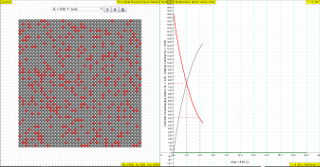Intro Page Designed by Fu-Kwun Hwang http://www.phy.ntnu.edu.tw/ntnujava/ http://weelookang.blogspot.sg/2015/12/ejss-radioactive-decay-model.html

### Translations

Code Language Translator Run### Software Requirements

SoftwareRequirements

 Android iOS Windows MacOS with best with Chrome Chrome Chrome Chrome support full-screen? Yes. Chrome/Opera No. Firefox/ Samsung Internet Not yet Yes Yes cannot work on some mobile browser that don't understand JavaScript such as..... cannot work on Internet Explorer 9 and below

### CreditsFu-Kwun Hwang - Dept. of Physics, National Taiwan Normal Univ.; lookang

### end faq

http://iwant2study.org/lookangejss/06QuantumPhysics_20nuclear/ejss_model_decaychangeNwee/decaychangeNwee_Simulation.xhtml

### Theory

The law of radioactive decay predicts how the number of the not decayed nuclei of a given radioactive substance decreases in the course of time. The GREY circles of this simulation symbolize 100 (variable selectable up 400 or 1024) to  atomic nuclei of a radioactive substance whose half-life period (T1/2) amounts to 0.1 to 3.0 seconds. The scientfic graph on the right, in number of radioactive atoms versus time, RED trail represents represents the Number of radioactive atoms, N₁ not yet decayed nuclei at a given time t, predicted by the following law:

N1   =   N0 e -(ln2/T1/2)) t

when you play with the show model option, show me N1= No*exp(-ln(2)/T1/2*t)

similarly it is possible to also predict the number of decayed atoms using the formula

N2  =   N0 -N0 e -(ln2/T1/2)) t

when you play with the show model option, show me N2  = No-No*exp(-ln(2)/T1/2*t)

in the option both2 when selected, can display the rate of radioactive atoms versus time,

dN = pN0 e -(ln2/T1/2)) t

when you play with the show model option, show me dN = p*N0*exp(-ln(2)/T1/2*t)

the terms use are

N1 .... number of the not decayed nuclei

N2 .... number of the decayed nuclei

N0 ... number of the initially existing nuclei at time, t = 0
t .... time
T1/2 ....  is the time taken for the activity of a given amount of a radioactive substance to decay to half of its initial value

λ,  or sometimes also known as "lambda" the inverse of the mean lifetime, sometimes referred to as simply decay rate.

p  is probability of decaying where 0 means no chance at all, 1 is 100% chance of decaying

Formula that related some of these terms are

T1/2 = ln(2)/λ

$$\lambda = \frac{p}{dt}$$

The Ordinary Differential equations used is

$$\frac{dN_{1}}{dt} = - \lambda_{1}N_{1}$$

### Versions

1. http://weelookang.blogspot.sg/2016/02/a-comparison-of-2-javascript-nuclear.html comparison of 2 HTML5 versions
3. http://www.phy.ntnu.edu.tw/ntnujava/index.php?topic=194.0 by Fu-Kwun Hwang original Java version
4. http://www.opensourcephysics.org/items/detail.cfm?ID=10576 by Wolfgang Christian similar Java version

### Other Resources

1. http://www.opensourcephysics.org/items/detail.cfm?ID=13978&S=7 Two-State Nuclear Decay JS Model written by Wolfgang Christian
2. http://www.walter-fendt.de/html5/phen/lawdecay_en.htm by Walter fendt

# Testimonials (0)

There are no testimonials available for viewing. Login to deploy the article and be the first to submit your review!